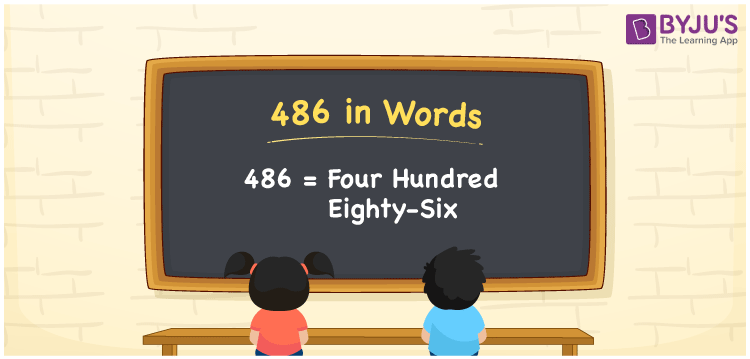# 486 in Words

486 in words is written as Four hundred eighty-six. In both the International System of Numerals and the Indian System of Numerals, 486 is written as Four hundred eighty-six. The number 486 is a Cardinal Number as it represents some quantity. For example, “the area of this land is 486 square feet”.

 486 in Words Four hundred eighty-six Four hundred eighty-six in Number 486

## 486 in English Words

We write 486 in English Words using the letters of the English alphabet. Therefore, we read 486 in English as “Four hundred eighty-six.”## How to Write 486 in Words?

To write 486 in words, we shall use the place value chart. In the place value chart, write 4 in the hundreds, 8 in the tens, and 6 in the ones, respectively. Now let us make a place value chart to write the number 486 in words.

 Hundreds Tens Ones 4 8 6

Thus, we can write the expanded form as

4 × Hundred + 8 × Ten + 6 × One

= 4 × 100 + 8 × 10 + 6 × 1

= 400 + 80 + 6

= 486

= Four hundred eighty-six.

486 is a natural number, the successor of 485 and the predecessor of 487.

486 in words – Four hundred eighty-six

• Is 486 an odd number? – No
• Is 486 an even number? – Yes
• Is 486 a perfect square number? – No
• Is 486 a perfect cube number? – No
• Is 486 a prime number? – No
• Is 486 a composite number? – Yes

## Frequently Asked Questions on 486 in Words

Q1

### How to write 486 in words?

486 in words is written as Four hundred eighty-six.
Q2

### How to write 486 in the International and Indian System of Numerals?

In both, the system of numerals, 486 in words, is written as Four hundred eighty-six.
Q3

### What is the preceding number of 486?

The number that precedes 486 is 485.